Question

# Friction is the force that a. is the same as the normal force b. opposes relative...

Friction is the force that

a. is the same as the normal force

b. opposes relative motion between objects in contact

c. is always perpendicular to the surface

d. is the same as weight

This question is based on the concept of

1. Free body diagram

2. Friction force

3. Direction of friction force

The detailed solution is described below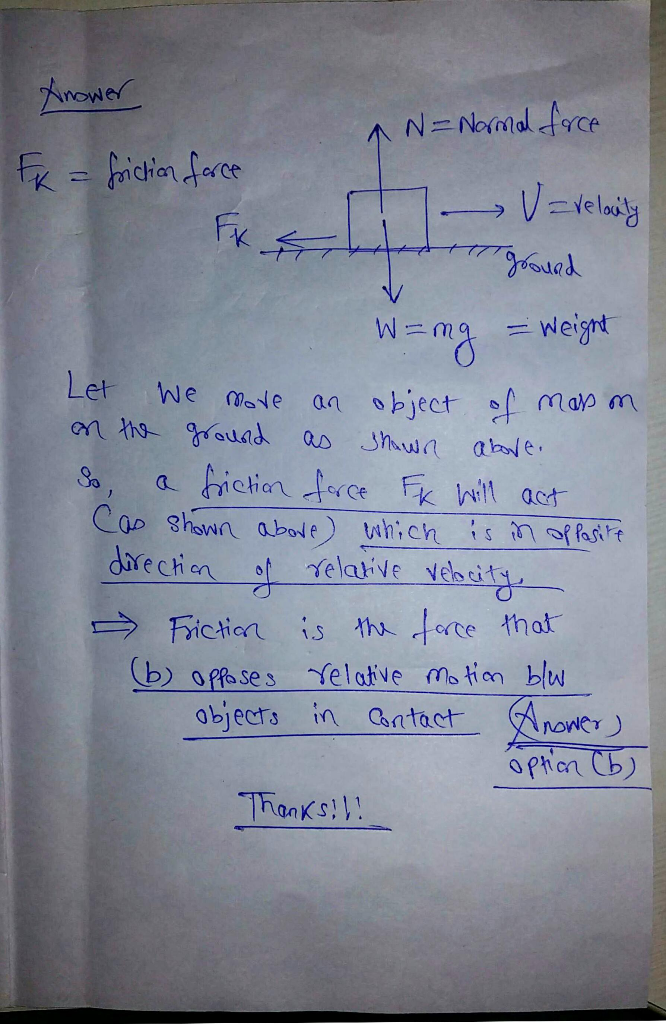THANKS

#### Earn Coins

Coins can be redeemed for fabulous gifts.

Similar Homework Help Questions
• ### The coefficient of kinetic friction between two materials depends on Select one: a. the normal force...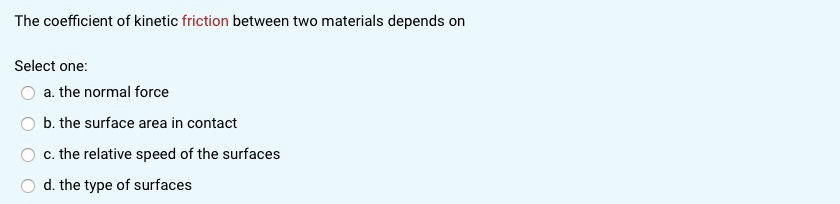The coefficient of kinetic friction between two materials depends on Select one: a. the normal force b. the surface area in contact c. the relative speed of the surfaces d. the type of surfaces

• ### Which statement about friction and normal force is true? a. Kinetic friction is always in the...

Which statement about friction and normal force is true? a. Kinetic friction is always in the direction of the normal force. b. Static and kinetic friction do not depend on the normal force. c. Static and kinetic friction forces are linearly related to the normal force. d. Static friction is always in the direction of the normal force.

• ### Static friction opposes gravity, which acts to pull a wood block down an inclined plane. What...

Static friction opposes gravity, which acts to pull a wood block down an inclined plane. What is the total value of the gravitational force (Fg) and the force due to friction (Ff) in Newton’s if the wood block is sitting on a flat surface? (A) 0 N (B) 9.8 N (C) 4.9 N (D) 2.8 N

• ### (Newton's laws/gravitation force/normal force/friction force, 4/ea.) A 60-N force pushes two objects next to each other...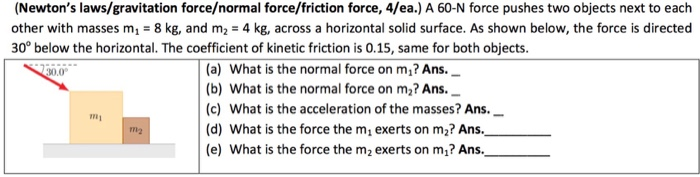(Newton's laws/gravitation force/normal force/friction force, 4/ea.) A 60-N force pushes two objects next to each other with masses m_1 = 8 kg, and m_2 = 4 kg, across a horizontal solid surface. As shown below, the force is directed 30 degree below the horizontal. The coefficient of kinetic friction is 0.15, same for both objects. What is the normal force on m_1? What is the normal force on m_2? What is the acceleration of the masses? What is the force...

• ### Although friction is a complicated force, many aspects of it can be described with a simple model.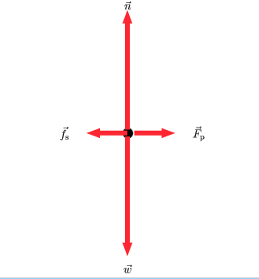Tactics Box 5.1 Working with Friction ForcesLearning Goal:To practice Tactics Box 5.1 Working with friction forces.Although friction is a complicated force, many aspects of it can be described with a simple model. This Tactics Box presents the conditions under which these models are valid and outlines the essential steps in solving friction problems.TACTICS BOX 5.1 Working with friction forces1. If the object is not moving relative to the surface it's in contact with, the friction force is static friction. Draw...

• ### Work Done by Normal Force Consider an object sliding along the surface shown below. Choose and...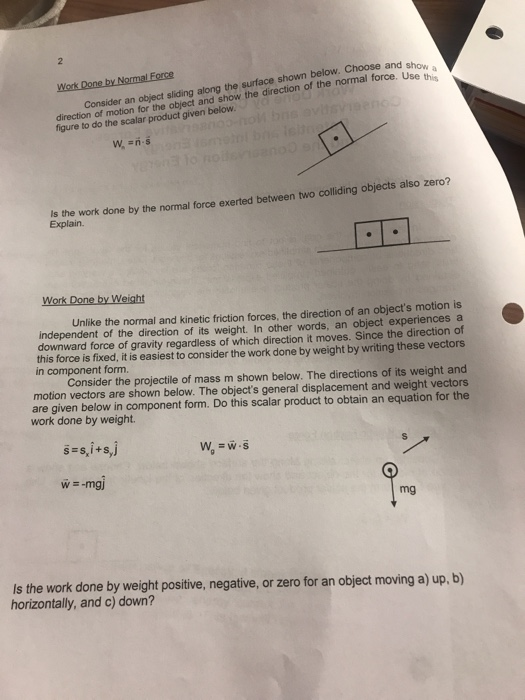Work Done by Normal Force Consider an object sliding along the surface shown below. Choose and show a direction of motion for the object and show the direction of the normal force. Use this figure to do the scalar product given below. oM bns av W, n-s Is the work done by the normal force exerted between two colliding objects also zero? Explain. Work Done by Weight Unlike the normal and kinetic friction forces, the direction of an object's motion...

• ### When an object rests on a surface, there is always a force perpendicular to the surface; we call this the normal force,...When an object rests on a surface, there is always a force perpendicular to the surface; we call this the normal force, denoted by . The two questions to the right will explore the normal force. figure A figure B A man attempts to pick up his suitcase of weight by pulling straight up on the handle. (Part A figure) However, he is unable to lift the suitcase from the floor. Which statement about the magnitude of the normal force...

• ### Which of the following forces require contact? Select all that apply. Kinetic friction force Normal force...

Which of the following forces require contact? Select all that apply. Kinetic friction force Normal force Spring force Static friction force Tension force Weight force

• ### A small block of mass m1=4 kg is placed at rest on a larger block of mass m2=6kg. The coefficient of friction between the two block is ų=0.3. And the horizontal surface is smooth. A constant force F is applied on the block. The situation is given in the f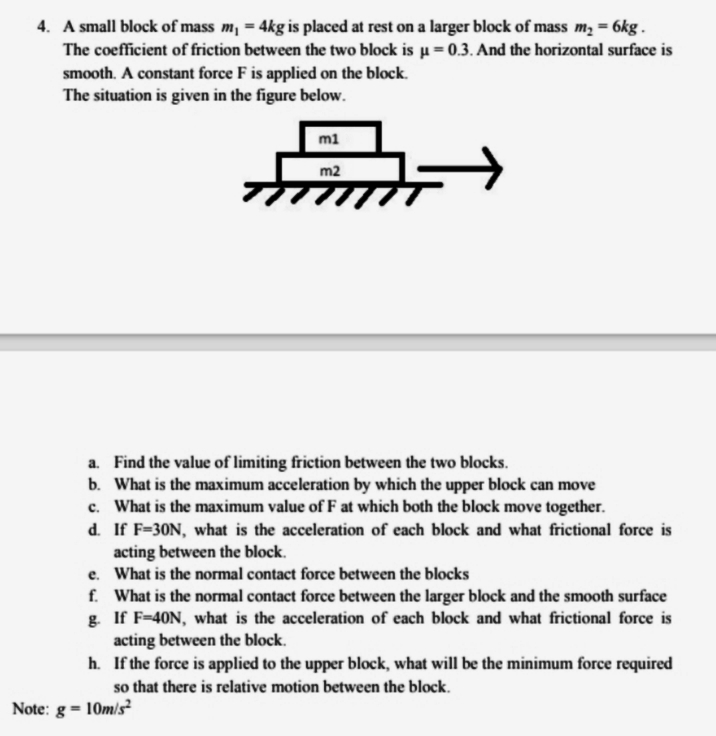4. A small block of mass $m_{1}=4 k g$ is placed at rest on a larger block of mass $m_{2}=6 \mathrm{kg}$. The coefficient of friction between the two block is $\mu=0.3 .$ And the horizontal surface is smooth. A constant force $\mathrm{F}$ is applied on the block.  The situation is given in the figure below. a. Find the value of limiting friction between the two blocks. b. What is the maximum acceleration by which the upper block can move c....

• ### Q1: When two objects slide against oneanother, which of the following statements about the force offriction...

Q1: When two objects slide against oneanother, which of the following statements about the force offriction between them is true? Themagnitude of the frictional force is always equal to . Themagnitude of the frictional force is always less than . Themagnitude of the frictional force is determined by other forces onthe objects so it can be either equal to or less than . Q2: When two objects are in contact with norelative motion, which of the following statements about thefrictional...